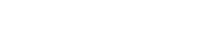# ISA Certified Control Systems Technician (CCST) program

• March 31, 2020
• CCST

Certified Control System Technicians (CCSTs) calibrate, document, troubleshoot, and repair/replace instrumentation for systems that measure and control level, temperature, pressure, flow, and other process variables.

### CCST question

In a system where flow is measured with an orifice plate, the flow is inferred from a change in pressure as the fluid pass through the orifice. This change in pressure is caused by the increase in fluid velocity through the orifice hole. The principle that describes this change in pressure due to the increase in velocity is called:

A. Ohm’s law
B. Bernoulli’s principle
C. orifice beta ratio principle
D. Pascal’s law

The answer is B, Bernoulli’s principle. In the diagram below,

Volumetric Flow = A1 × V1 = A2 × V2

The above continuity equation coupled with Bernoulli’s equation yields the relationship between the pressure at locations 1 and 2:

Flow is proportional to √ (P1 – P2)

Where the pressure at P2 < P1 and the velocity at V2 > V1.

Reference: Goettsche, L.D. (Editor), Maintenance of Instruments and Systems, Second Edition, ISA, 2005.